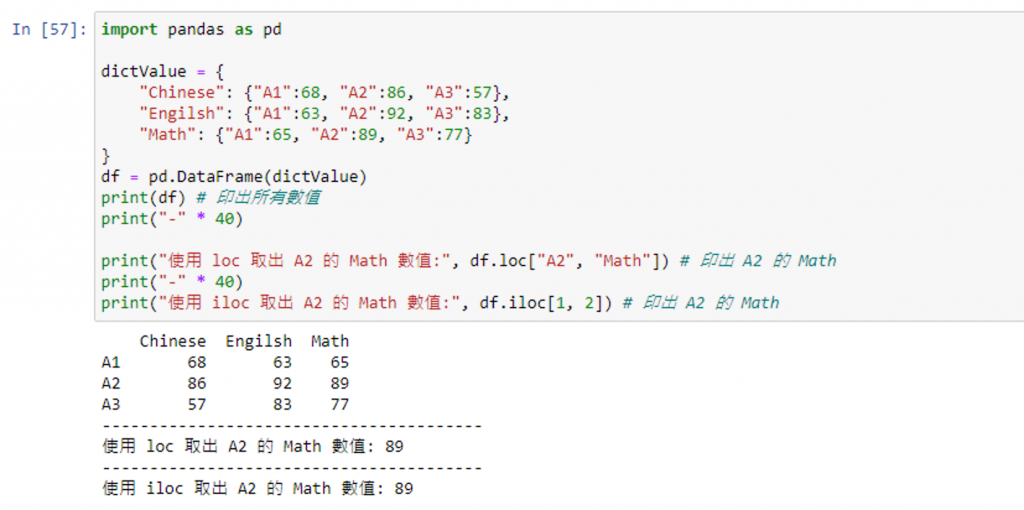#DAY 28
1
Software Development

## [2020鐵人賽Day28]糊裡糊塗Python就上手-Pandas的觀念與運用(上)

### What is Pandas?

Pandas 是基於 Numpy 開發的模組，該模組是為了解決結構化資料分析而創建的，Pandas納入了大量函數和一些標準的資料模型，用於資料挖掘和資料分析，同時也提供數資料清洗功能

### 事前準備

#### 安裝與導入 Pandas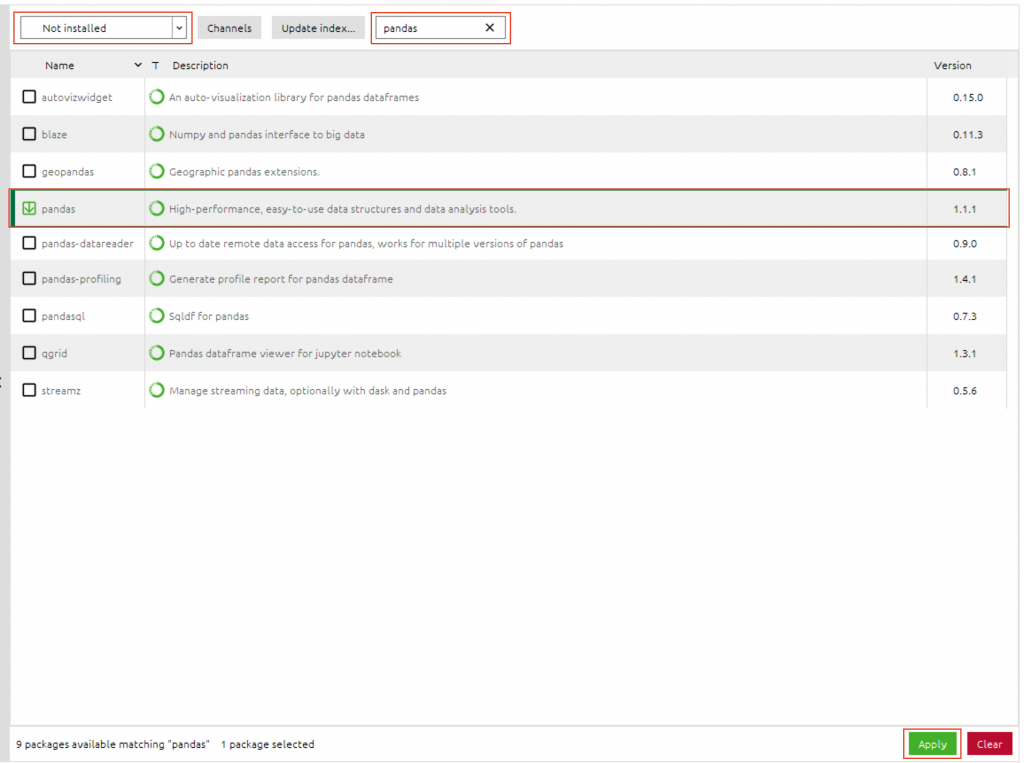``````pip install pandas
``````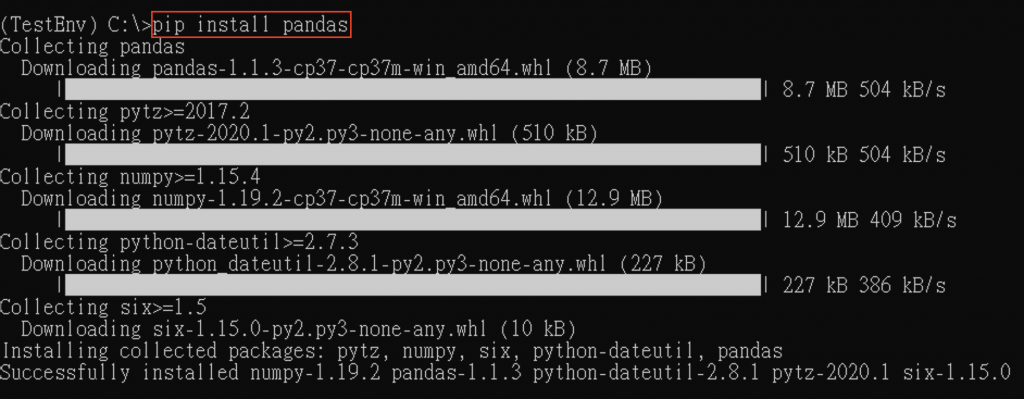``````import pandas as pd
``````

### Pandas 觀念介紹 & 實際體驗

#### Pandas 資料結構

Series|有索引值的一維資料陣列|pd.Series(資料來源[, index = 自訂索引])
DataFrame|有索引值與欄標籤的二維資料集|pd.DataFrame(資料來源[, index = 自訂索引, columns = 欄位])

#### Series 實際體驗

``````import pandas as pd

# 情境一
listValue = ['Apple', "Banana", "Cherry"]
se = pd.Series(listValue)
print(se) # 印出所有內容
print(se.values) # 印出數值
print(se.index) # 印出索引

print("-" * 20)

# 情境二
listValue2 = ['Apple', "Banana", "Cherry"]
se2 = pd.Series(listValue2, ["v1", "v2", "v3"])
print(se2) # 印出所有內容
print(se2.index) # 印出索引
``````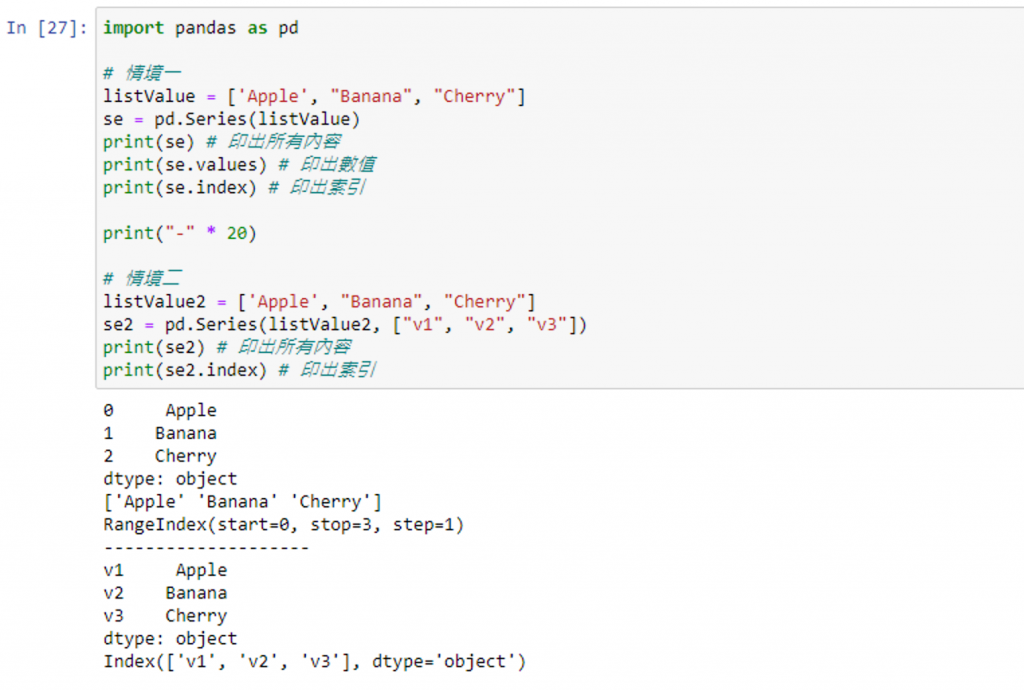``````import pandas as pd

# 情境三
dictValue = {
"Engilsh": 'mediocre',
"Math": 'excellent'
}

se = pd.Series(dictValue)
print(se) # 印出所有內容
print("-" * 20)
print(se.values) # 印出數值
print("-" * 20)
print(se.index) # 印出索引
print("-" * 20)
print(se) # 印出指定索引位置的數值
print("-" * 20)
print(se["Engilsh"]) # 印出指定索引位置的數值
print("-" * 20)
print(se[[0, 2]]) # 印出指定索引位置的數值
``````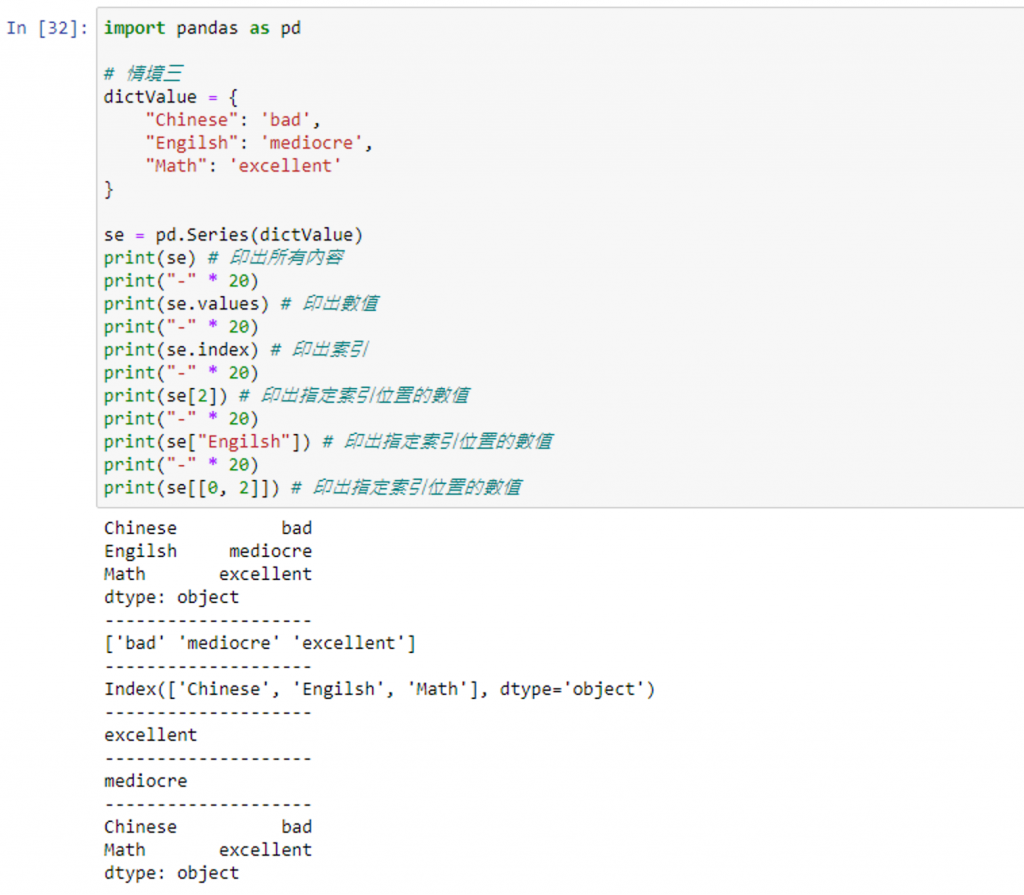#### DataFrame 實際體驗

index 索引是第一列的值，column 是第一欄的欄位名稱(若未給予將會預設給予由 0 開始的整數串列)

``````import pandas as pd

listValue = [
["Apple", "Banana", "Cherry"],
["Grape", "Lemon", "Orange"],
["Kiwi", "Pineapple", "Watermelon"]
]

# 情境一：未給予 index 值與 column值
df = pd.DataFrame(listValue)
print(df)

print("-" * 40)

# 情境二：給予 index 值，未給予 column值
df2 = pd.DataFrame(listValue, index=[1, 2, 3])
print(df2)

print("-" * 40)

# 情境三：給予 index 值與 column值
df3 = pd.DataFrame(listValue, index=[1, 2, 3], columns=["v1", "v2", "v3"])
print(df3)
``````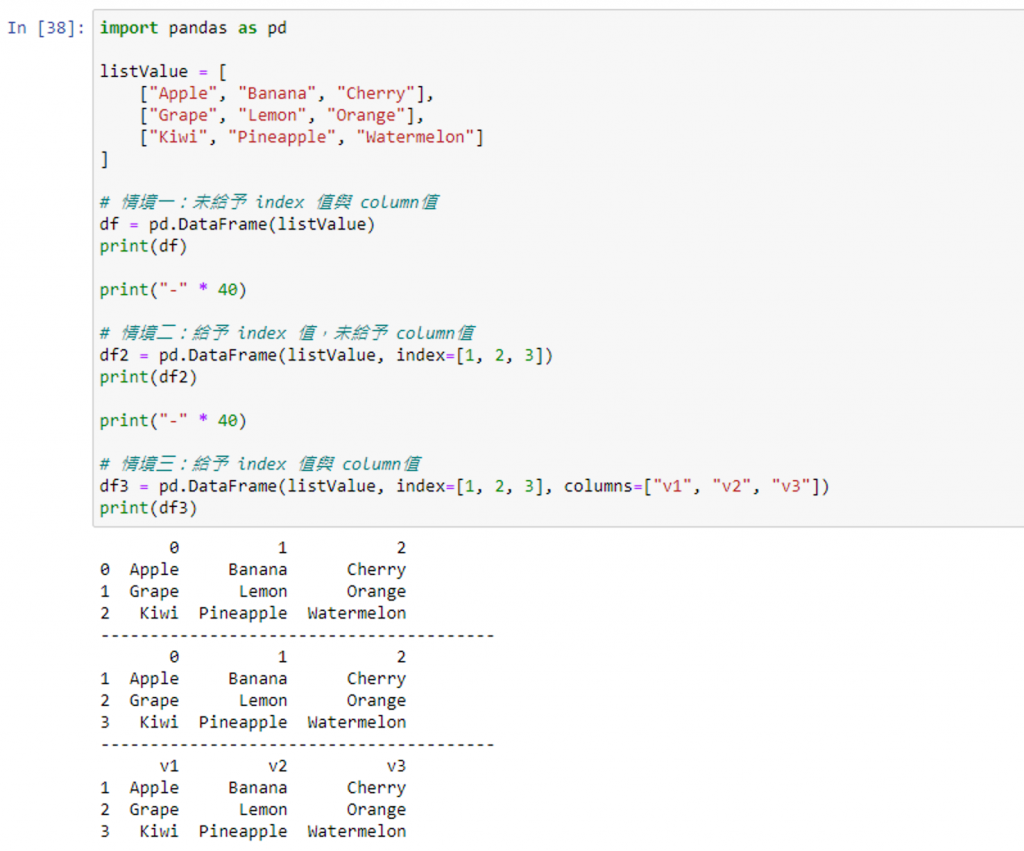``````import pandas as pd

# 情境四
dictValue = {
"Chinese": {"A1":68, "A2":86, "A3":57},
"Engilsh": {"A1":63, "A2":92, "A3":83},
"Math": {"A1":65, "A2":89, "A3":77}
}
df = pd.DataFrame(dictValue)
print(df)
``````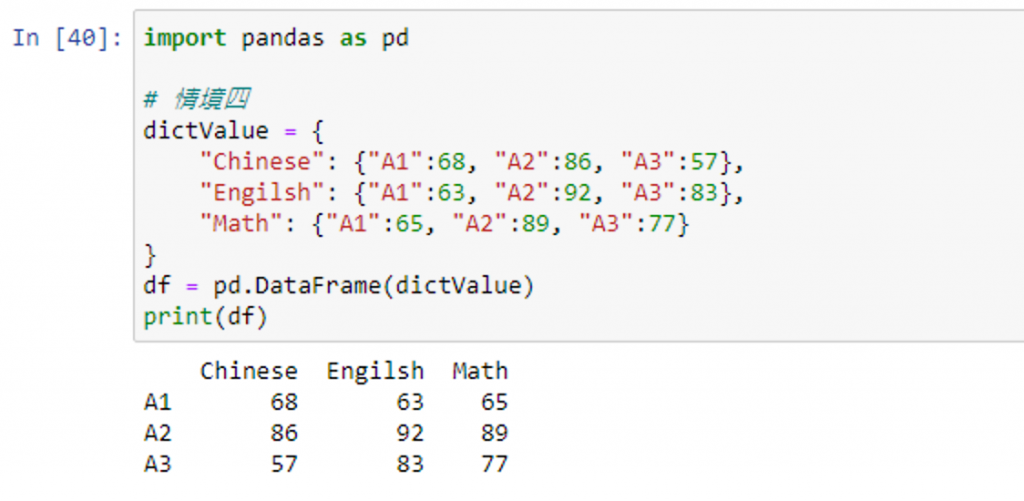#### 取得 DataFrame 的相關數值

df["欄位名稱"] 使用欄位名稱取值
df[["欄位名稱1", "欄位名稱2"...]] 多個欄位名稱取值
df[df["欄位名稱"] 條件式] 以條件式去判定指定的欄位是否符合條件，若是符合則為True，不符合則為False，再藉由判斷後的回傳值印出符合的所有數值
df["欄位名稱"].values 僅取出指定欄位內的數值
df.values[索引位置] 取出指定索引的數值
df.columns 取出欄位名稱
df.indes 取出 index
df.describe() 描述性統計值

``````import pandas as pd

dictValue = {
"Chinese": {"A1":68, "A2":86, "A3":57},
"Engilsh": {"A1":63, "A2":92, "A3":83},
"Math": {"A1":65, "A2":89, "A3":77}
}
df = pd.DataFrame(dictValue)
print(df) # 印出所有數值

print("-" * 40)

print("取出 Chinese 的相關數值:\n", df["Chinese"]) # 印出 Chinese 的相關數值
print("-" * 40)
print("取出 Chinese Math 的相關數值:\n", df[["Chinese", "Math"]])# 印出 Chinese Math 的相關數值
print("-" * 40)
print("取出以 Chinese 為基準，取其中及格(>=60)的所有相關數值:\n", df[df["Chinese"] >= 60]) # 印出以 Chinese 為基準，取其中及格(>=60)的所有相關數值
print("-" * 40)
print("僅取出 Chinese 的數值:\n", df["Chinese"].values) # 印出僅取出 Chinese 的數值部分
print("-" * 40)
print("僅取出 A1 的 Engilsh 的數值:\n", df.values) # 印出僅取出 A1 的 Engilsh 的數值
print("-" * 40)
print("取出欄位名稱:", df.columns) # 印出欄位名稱
print("-" * 40)
print("取出 index:", df.index) # 印出 index
print("-" * 40)
print("印出描述性統計值:\n", df.describe()) # 印出描述性統計值
``````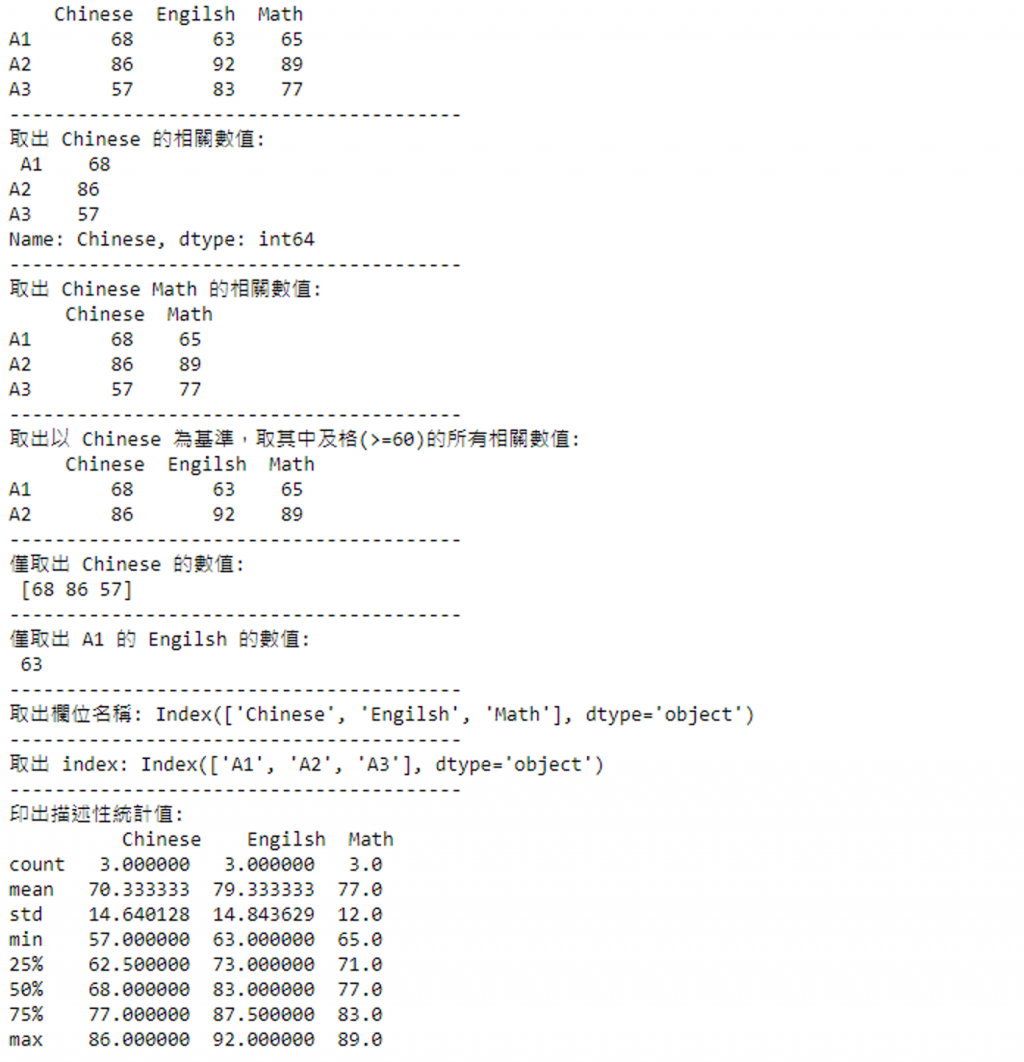df.loc["索引名稱", "欄位名稱"] 以索引名稱及欄位名稱取得資料
df.iloc["索引編號", "欄位編號"] 以索引編號及欄位編號取得資料(資料起始為0)
df.tail(n) 取得最後面的指定筆數資料，n 為選擇性填寫參數，若不填寫則取得最後面 5 筆資料

``````import pandas as pd

dictValue = {
"Chinese": {"A1":68, "A2":86, "A3":57},
"Engilsh": {"A1":63, "A2":92, "A3":83},
"Math": {"A1":65, "A2":89, "A3":77}
}
df = pd.DataFrame(dictValue)
print(df) # 印出所有數值
print("-" * 40)

print("使用 loc 取出 A2 的 Math 數值:", df.loc["A2", "Math"]) # 印出 A2 的 Math
print("-" * 40)
print("使用 iloc 取出 A2 的 Math 數值:", df.iloc[1, 2]) # 印出 A2 的 Math
``````